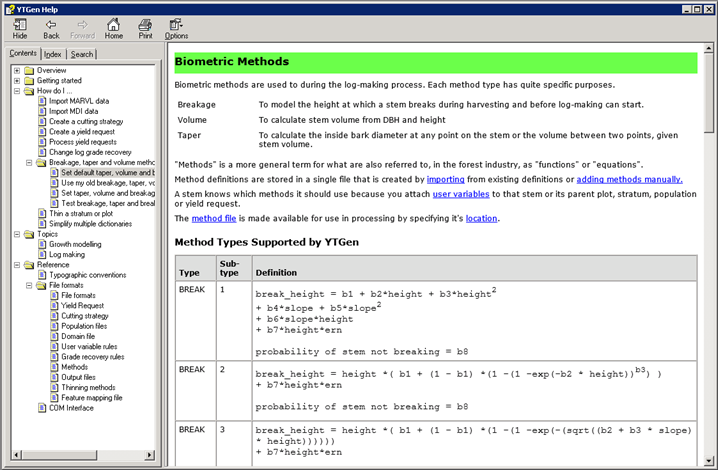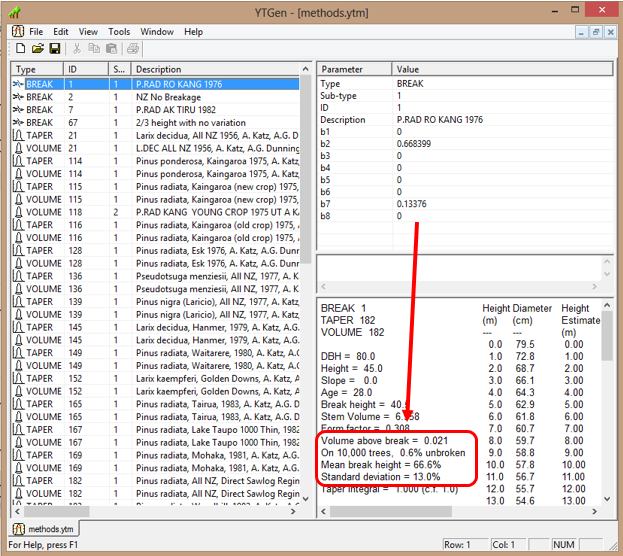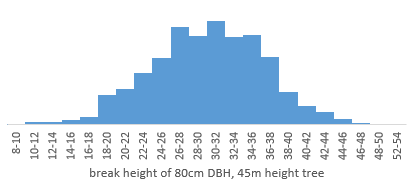+64 7 350 3209 +61 2 8011 3645 info@interpine.co.nz
Select Page

New users often ask, what biometric methods are supported by YTGEN? In the forest industry most people consider these are functions or equations used to model the shape, volume, and inside bark diameter at any point on the stem. These are used in the log-making process to emulate felling practices like stem breakage, and to calculate stem volumes and allow bucking model to fit log products into their diameter specifications.

So what does YTGEN consider to be a biometric method?

In YTGEN these are generalized in the term “Methods” and are stored inside a Method file (*.YTM). Each method type has quite specific purposes. For example a “breakage method” will model the height at which a stem breaks during harvesting and before log-making can start. A “volume method” will calculate stem volume from DBH and height. A “taper method” will calculate the inside bark diameter at any point on the stem or the volume between two points, given stem volume.

So What Type of Methods are Supported by YTGen?

You can define your own methods using the following common functional forms shown in table 1 and defined in table 2. Please note this is not a fixed list and just the current accepted method functional definition forms as of August 2013. Silmetra are adding to this list all the time when new forms become useful for users. However even with the forms shown below this provides resource foresters a wide scope for developing and implementing the right function for their own needs.

Table 1 – Method Definitions in YTGEN

 Type Sub-type Definition BREAK 1 break_height = b1 + b2*height + b3*height2 + b4*slope + b5*slope2 + b6*slope*height + b7*height*ern probability of stem not breaking = b8 BREAK 2 break_height = height *( b1 + (1 – b1) *(1 -(1 -exp(-b2 * height))b3) ) + b7*height*ern probability of stem not breaking = b8 BREAK 3 break_height = height *( b1 + (1 – b1) *(1 -(1 -exp(-(sqrt((b2 + b3 * slope) * height)))))) + b7*height*ern probability of stem not breaking = b8 TAPER 1 dib2 = volume/(k * height) * ( b1*X + b2*X2 + b3*X3 + b4*X4 + b5*X5 + b6*Xb7 + b8*Xb9 ) TAPER 9 dib2 = dob2 * ( b7 + b8*X + b9*Xb10) where: dob2 = ((dbh*height)/(height-bh))2 * (bc*Xb1 + b2*Xb3 + b4*Xb5 ) bc = ( (1 – bh/height)2 – b2*(1 – bh/height)b3 – b4*(1 – bh/height)b5 ) / (1 – bh/height)b1 TAPER 16 dib2 = volume/(k * height) * ( y + 2*X ) y =  bp1 * (3*X2 – 2*X) + b4 * dbh * (X – b5)2 * I1 + bp2 * dbh * (X – b6)2 * I2 bp1 = b1 + b2*dbh/height + b3*dbh/age bp2 = -b4 / ((b6-1) / (b5-1))3 I1 = 1 for x >= b5 0 for x < b5 I2 = 1 for x >= b6 0 for x < b6 Constraints: 0 < b5 <= 1.0 0 < b6 <= 1.0 TAPER 42 X >=  (1.0 – maxht): dib2 = volume/(k * height) * ( f(X)/X + d(X)*ln(X)) + Xf(X) ) where: f(X) = b1 + b2*X + b3*X2 + b4*X3 d(X) = b2 + 2*b3*X + 3*b4*X2 X < (1.0 – maxht): dib2 based on conic projection from X = (1.0 – maxht) TAPER 101 “Trigonometric Variable-Form Taper Equations for Australian Eucalypts”, Huiquan Bi, Forest Science 46(3) 2000, pp 397-409 The parameters a1-a7 that are used in YTGen have the same names and meaning as those in Equation 7 and Table 1 of the report. The parameters b0-b3 are used for calculating under-bark diameter at breast height and have the same names and meaning as those in Equation 9 and Table 2. bh allows an explicit specification of breast height (m) The Bi trigonometric variable-form taper function does not require, and will not use, a tree volume function. Volumes are calculated by numerical integration of diameter squared. TAPER 102 “A variable exponent taper equation”, A. Kozak, Canadian Journal of Forest Research, 18, 1988, pp. 1363-1368 The parameters a0-a2, b1-b5 and p that are used in YTGen have the same names and meaning as those in Table 5 and Equations 7 and 8 of the report. The Kozak variable exponent taper function does not require, and will not use, a tree volume function. Volumes are calculated by numerical integration of diameter squared. TAPER 103 “Flexible taper equation for site-specific management of Pinus radiata in New South Wales”, Huiquan Bi, Forest Ecology and Management 148 (2001) 79-91 The parameters a1-a7 that are used in YTGen have the same names and meaning as those in Equations 1 and 2 and Table 2 of the report. The parameters b0-b4 are used for calculating under-bark diameter at breast height and have the same names and meaning as those in Equations 3 and 9. bh allows an explicit specification of breast height (m) Sub-type 103 is the same as sub-type 101 with the addition of an extra bark-thickness term. The Bi trigonometric variable-form taper function does not require, and will not use, a tree volume function. Volumes are calculated by numerical integration of diameter squared. TAPER 105 “Segmented Polynomial Regression Applied to Taper Functions”, Timothy A. Max and Harold E Burkhart, Forest Science, volume 22, number 3, 1976, pp. 283-289 Model 4, which is the quadratic-quadratic-quadratic model. See Equation 4, p 285 and Table 3, p 288 The parameters a41 and a42 in YTGen correspond to alpha41 and alpha42 in Equation 4 the report. The parameters b41, b42, b43 and b44 in YTGen correspond to beta41 to beta44 in Equation 4 the report. This taper function does not require, and will not use, a stem volume function. Volumes are calculated by integration of the taper function. TAPER 107 The cubic tree taper model from “A cubic tree taper model”, Adrian Goodwin, Australian Forestry, Vol. 72, No. 2, pp. 87-98. The parameters delta10 – delta42 are the second-stage parameters from Tables 3 and 4 of the report. The parameter breast_height is the height constraint of the model, equivalent to h1 in equation 1b. CAUTION: This is an under-bark function. It makes no allowance for bark-thickness and will over-estimate volume when dbh is an over-bark diameter. VOLUME 1 volume = dbhb1 * (height2/(height-bh))b2 * exp(b3) + b4 VOLUME 2 volume = b1 + b2*dbh2 * height/10 000 + b3*dbh2/10 000 + b4*height + b5*dbh*height/10 000 VOLUME 3 log(volume) = b1 * log(dbh) + b2 * log(height) + b3 * dbh2 /10 000 + b4 VOLUME 4 volume = b1 * dbhb2 * heightb3 VOLUME 16 volume = exp( b1 + b2*log(dbhib) + b3*log(height) + b4*dbh/height + b5*dbh/age ) where: dbhib = dbh *(1 – exp(b6 + b7 * (1 – bh/height)9 + b8*dbh + b9*height/dbh VOLUME 17 volume = b1 * dbhb2 * THb3 where: TH = top height of plot containing stem, the mean height of the largest 100 stems per hectare by dbh. VOLUME 101 volume = exp( b1 + b2 * log(dbh) + b3 * log(height) + b4 * log(age) )

Table 2 – Definitions for variables found in Table 1

 Term Definition height Stem height (m) slope absolute value of ground slope in degrees ern a random number generated from a normal distribution with mean=0.0 and standard deviation=1.0 dbh stem over-bark diameter at breast height (cm) dib stem inside-bark diameter (cm) bh breast height (m). This is a model parameter rather than an input. volume stem volume inside bark (m3) h Stem position as distance from ground level (m) along stem X (distance from tip of tree (m)) / height = (height – h)/height k PI / 40 000 b1-b9 Parameters, the values of which are user-supplied. dbhib stem diameter inside bark at breast height (cm) age plot age (years) log(x) natural logarithm (base e)

Find out more?

You can find out more about using methods in the YTGEN Help file.Figure 1 YTGEN Help file on Biometric Methods

Example of a Breakage Model Implemented

One of most commonly used stem breakage models used in P.radiata is what most New Zealand resource foresters call “breakage 1”.   This was developed in 1976 from stems felled in a study in Kaingaroa Forest in the Rotorua wood supply district.   It uses breakage subtype 1, with coefficient variables b2=0.668399 and b7=0.13376, with all remaining set to 0.

So what does this mean?

Well using the “method test” feature of YTGEN you can quickly set a test stem and complete a simulation of breaking 10,000 trees and get an idea of what the breakage model does.   So in Figure 2 below you can see 0.6% trees will remain unbroken, with a mean break height of 0.66% of the tree height and the standard deviation of this mean break height being 13%.   So quickly you can see what the effect of the coefficients b2 and b7, these being the mean break height as a % of the tree height (b2*height) and b7 creating a normal distribution of break heights around the mean using a random number generated from a normal distribution with mean=0.0 and standard deviation=1.0  (b7*height*ern).   Ofcourse setting most of the other coefficients means they are not used (0*x=0), except b8 which by definition has meant that the probablity of the stem not breaking has been set to 0% (except for influence of the ern = 1.0, which would mean the odd stem does not break, hence 0.6% of stem remain unbroken in the test dataset).

You can graph this up yourself by using the “copy break heights to clipboard” feature available from the right click inside the test stem results below.  Doing this enables you to quickly paste these into EXCEL for example and look at break heights as shown in Figure 3.Figure 2 – example of the Method File view in YTGEN with a test stem using Breakage function 1Figure 3 – distribution of break heights from the test of 10,000 trees using breakage function 1